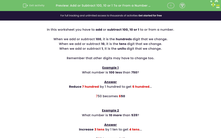# Add or Subtract 100, 10 or 1 To or From a Number (6)

In this worksheet, students add or subtract 100, 10 or 1 to or from a given number.Key stage:  KS 2

Curriculum topic:   Number: Number and Place Value

Curriculum subtopic:   Find 10 or 100 More or Less than a Given Number

Difficulty level:#### Worksheet Overview

In this worksheet you have to add or subtract 100, 10 or 1 to or from a number.

When we add or subtract 100, it is the hundreds digit that we change.

When we add or subtract 10, it is the tens digit that we change.

When we add or subtract 1, it is the units digit that we change.

Remember that other digits may have to change too.

Example 1

What number is 100 less than 750?

Reduce 7 hundred by 1 hundred to get 6 hundred...

750 becomes 650

Example 2

What number is 10 more than 539?

Increase 3 tens by 1 ten to get 4 tens...

539 becomes 549

Example 3

What number is 1 less than 539?

Reduce 9 units by 1 unit to get 8 units...

539 becomes 538

Example 4

What number is 10 less than 405?

Here we have to be careful, because we can't reduce the tens digit by 1.

So include the hundreds digit.

Think of it as reducing 40 tens by 1 ten to get 39 tens.

405 becomes 395

### What is EdPlace?

We're your National Curriculum aligned online education content provider helping each child succeed in English, maths and science from year 1 to GCSE. With an EdPlace account you’ll be able to track and measure progress, helping each child achieve their best. We build confidence and attainment by personalising each child’s learning at a level that suits them.

Get started